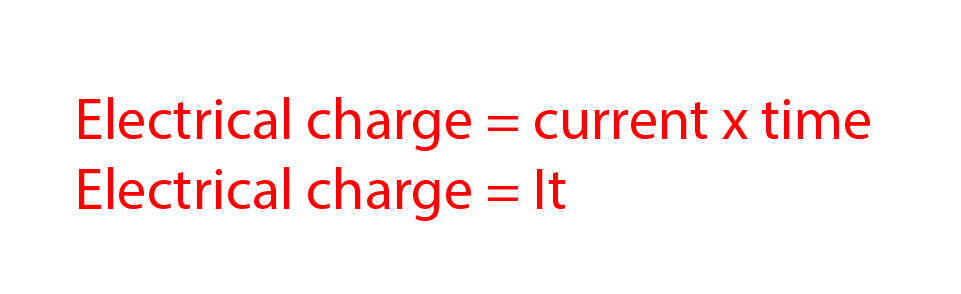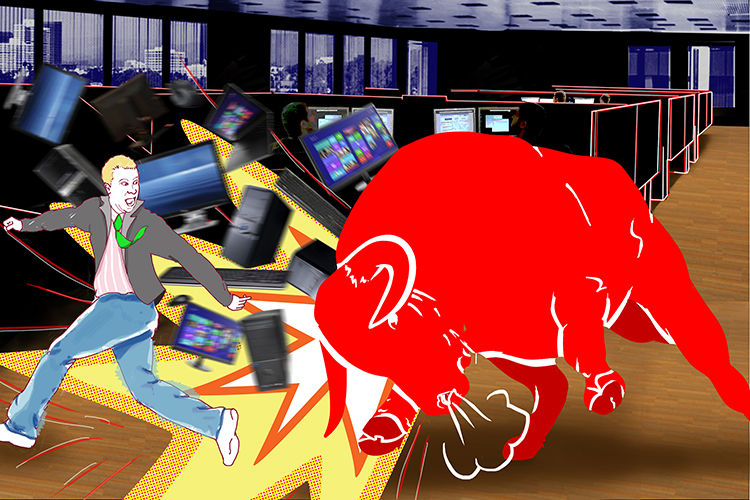# Electrical charge

Electrical current within a circuit is the flow of electrical charge over time. The formula for calculating electrical charge is:A bull charges into the IT department.

NOTE:

You may see this formula written as Q = It

Electrical charge is measured in coulombs (C)

Current is measured in amps (A)

And time is measured in seconds (s)

The internationally agreed symbols for this formula are:

Q = Charge

I = Current

t = time

To remember that charge is measured in coulombs think of this image:The bull charges into columns (Coulombs) of computers.

Example 1

A current of 12A flows for 20 minutes into an electric cooker. How much charge has the cooker used?

E\l\e\c\t\rical\ ch\a\r\g\e = Currentxxtime

But don’t forget that the time has to be converted to the standard unit.
So 20\ m\i\n\u\tes = 20xx60 = 1200\ s\e\c\o\nds Therefore:

E\l\e\c\t\rical\ ch\a\r\g\e = 12xx1200=14400\ co\u\l\ombs

Example 2

If 18400 C of charge flows through an air conditioning unit every hour, what current does it draw?

E\l\e\c\t\rical\ ch\a\r\g\e = Currentxxtime

Divide both sides by time to make current the subject,

(E\l\e\c\t\rical\ ch\a\r\g\e)/(time) = (Currentxxcancel(time))/cancel(time)

Current=(E\l\e\c\t\rical\ ch\a\r\g\e)/(time)

The time must be converted from hours to the standard unit of seconds, so:

1\ hour = 60\ m\i\n\u\tes = 60xx60 = 3600\ s\e\c\o\nds

therefore:

Current=18400/3600=5.1\ amps